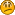# Cassini's AU

• 3 Replies
• 2590 Views#### ﮎingulaЯiτy

• Arbitrator
• Planar Moderator
• 9074
• Resident atheist.##### Cassini's AU
« on: October 06, 2008, 07:18:34 AM »
Astronomical Unit History:

"Tycho Brahe estimated the distance between the Sun and the Earth at 8 million kilometers (5 million miles). Later, Johannes Kepler estimated the AU was at 24 million kilometers (15 million miles). In 1672, Giovanni Cassini made a much better estimate by using Mars. By observing Mars from Paris and having a colleague, Jean Richer, also observe Mars at the same time in French Guiana in South America, Cassini determined the parallax of Mars. From that Cassini was able to calculate the distance from Earth to Mars, and then the distance from Earth to the Sun. Cassini calculated the AU to be at 140 million kilometers (87 million miles), which is lower, but very close to the modern day number."

Tom, I know you had an explanation for the AU being horribly wrong, but I forget it. To debate this, I need to know your side. You post way to much and say way too little regarding this for my searches to work. Stuff like, "AU's are overestimated" show up but not reasons.If I was asked to imagine a perfect deity, I would never invent one that suffers from a multiple personality disorder. Christians get points for originality there.#### Tom Bishop

• Flat Earth Believer
• 17521##### Re: Cassini's AU
« Reply #1 on: October 06, 2008, 10:25:56 AM »
The AU is wrong because it's derived by studying the position of the sun from two far off places on earth and assuming that it is a globe by accounting for its supposed curvature.

Each astronomer throughout history has measured distance of the Earth and Sun by using triangulation between two distant points on Earth, observing the Sun and measuring the angle of the sun in the sky in relation to the sea-level of the Earth. An astronomer could either triangulate the Sun's distance from the Earth to 93 million miles or 3,000 miles. It entirely depends on whether the earth appears as flat or round in the author's triangulation equations. Different angles mean different things on an RE and an FE.

For example, this link shows us how modern Round Earth science calculated the distance between the Earth and Sun using the transit of Venus. You will immediately notice that the equations are highly dependent on the assumption of a Round Earth.

If we take those same triangulation equations and use them under the assumption of a Flat Earth and do away with the compensation for the curvature of the Earth, assuming a flat surface, the final equation for the distance between the Earth and Sun becomes

rT = (PT / PV)2/3 (1 - eT cos ET) / (1 - eV cos Ev)

When we plug in the numbers from that link, the figure 'rT', the distance between the Earth and Sun, is equated out to an approximation close to 3,000 miles.

Finally, the angular size of the sun is 0.5°. Using this fact with a distance to the sun of 3000 miles, gives the sun's diameter: 32 miles.
« Last Edit: October 06, 2008, 10:30:56 AM by Tom Bishop »#### markjo

• Content Nazi
• The Elder Ones
• 39107##### Re: Cassini's AU
« Reply #2 on: October 06, 2008, 10:40:37 AM »
For example, this link shows us how modern Round Earth science calculated the distance between the Earth and Sun using the transit of Venus. You will immediately notice that the equations are highly dependent on the assumption of a Round Earth.

If we take those same triangulation equations and use them under the assumption of a Flat Earth and do away with the compensation for the curvature of the Earth, assuming a flat surface, the final equation for the distance between the Earth and Sun becomes

rT = (PT / PV)2/3 (1 - eT cos ET) / (1 - eV cos Ev)

When we plug in the numbers from that link, the figure 'rT', the distance between the Earth and Sun, is equated out to an approximation close to 3,000 miles.

Tom, did you actually plug in the numbers and do the math, or are you just assuming that they would give you the answer that you expect?
Science is what happens when preconception meets verification.
Quote from: Robosteve
Besides, perhaps FET is a conspiracy too.
Quote from: bullhorn
It is just the way it is, you understanding it doesn't concern me.#### cmdshft

• The Elder Ones
• 13129
• swiggity swooty##### Re: Cassini's AU
« Reply #3 on: October 06, 2008, 12:05:58 PM »
are you just assuming that they would give you the answer that you expect?

This.